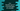# C++ program to find the sum of the first and last digits of a number## C++ program to find the sum of the first and last digits of a number:

In this post, we will learn how to find the sum of the first and the last digits of a number. For example, if the number is 2341, it will give us the sum of 2 and 1, i.e. 3.

The program will take the number as an input from the user and print the sum of the first and the last digits of that number.

With this program, you will learn how to use an empty for loop and how to get the first and the last digits of a number.

### Algorithm to use:

We will use the below algorithm to find the sum of the first and the last digits for a number:

• Take the number as input from the user.
• Find the last digit of the number by using the modulo operator. If you use number % 10, it will return the remainder if we divide the number by 10.
• For the first digit, we will intialize another variable with the value of the number and keep dividing it by 10 until we get a single digit. For example, if the number is 123, if we divide it by 10, it will be 12, then if we divide 12 by 10, it will be 1, which is the first digit.
• Once we get both of these digits, we can add these digits to find the sum.

### C++ program:

Let’s learn how to write the C++ program that finds the sum of the first and the last digits:

``````#include <iostream>
#include <string>
using namespace std;

int main()
{
int num, sum;

cout << "Enter a number: " << endl;
cin >> num;

int lastDigit = num % 10;
int firstDigit;

for (firstDigit = num; firstDigit > 10; firstDigit = firstDigit / 10)
;

sum = firstDigit + lastDigit;

cout << "The sum of the first and the last digits of " << num << " is : " << sum<< endl;

return 0;
}``````

Here,

• num and sum are two integer variables to hold the user input number and the final sum.
• It is asking the user to enter a number. It reads that number and stores in the num variable.
• The last digit of the number is calculated by using % 10. This value is stored in the lastDigit variable.
• The firstDigit variable is initialized to hold the first digit of the number.
• The empty for loop runs from firstDigit = num and it runs till the value of firstDigit is greater than 10, i.e. two digits. On each step, it divides the number by 10, i.e. it removes the last digit of the number. Once the loop will end, firstDigit will hold the first digit of the number.
• The value of sum is calculated by adding the first and the last digit of that number.

### Sample output:

If you run this program, it will print output as like below:

``````Enter a number:
1238
The sum of the first and the last digits of 1238 is : 9

Enter a number:
33349812
The sum of the first and the last digits of 33349812 is : 5``````

It will work only for two or more digit numbers. For a single digit number, it will fail. You can add a check to verify if the given number is single digit.

``````#include <iostream>
#include <string>
using namespace std;

int findSumFirstLast(int num)
{
if (num < 10)
{
return num;
}

int lastDigit = num % 10;
int firstDigit;

for (firstDigit = num; firstDigit > 10; firstDigit = firstDigit / 10)
;

return firstDigit + lastDigit;
}

int main()
{
int num;

cout << "Enter a number: " << endl;
cin >> num;

cout << "The sum of the first and the last digits of " << num << " is : " << findSumFirstLast(num) << endl;

return 0;
}``````

This program will work for both single and multiple-digit numbers.

``````Enter a number:
9989
The sum of the first and the last digits of 9989 is : 18

Enter a number:
9
The sum of the first and the last digits of 9 is : 9``````